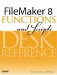# Minute()

Category: Time

 Syntax: Minute ( time )

Parameters:

timeAny valid time value or expression that returns a valid time value.

Data type returned: Number

Description:

The Minute() function returns an integer representing the number of minutes from the given time value.

The Minute() function always returns an integer in the range from 0 to 59. If you want the output of this function to be expressed always as a two-character string (for example, 07 instead of 7 when the time is 4:07 p.m.), use the following formula:

``` Right ("00" & Minute (time); 2)
```

Examples:

Function

Results

Minute ("10:45:20")

Returns 45.

Minute ("12:07 am")

Returns 7.

Minute (Get (CurrentTime))

Returns a value from 0 to 59.FileMaker 8 Functions and Scripts Desk Reference
ISBN: 0789735113
EAN: 2147483647
Year: 2004
Pages: 352